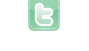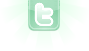# Button Function

1
2
How do you make the checks through the rows? I set up a variable for the rows (and columns) and made a for loop using it but I don't know how to make the remover move down the rows or how it removes the rows. I don't know what to do...
```public class RowRemover extends Actor
{
//Variables used
NumberWorld world = (NumberWorld) getWorld();
private int rows = 14;
private int cols = 9;

{
GreenfootImage img = new GreenfootImage(world.getWidth(), 2);
setImage(img);
}

public void go()
{
for(int i = 0; i < rows; i++)
{
//Skips rows that contain unsolved numbers
if(world.numbers.get(i).getValue() != 0)
{
continue;
}

//Whatever else I need here
}

world.removeObject(this);
}
}```
I believe lines 18 thru 24 can be replaced with:
```if (getintersectingObjects(Numbers.class).size() == getIntersetingObjects(Cover.class).size())
{
getWorld().removeObjects(getintersectingObjects(Actor.class));
}
setLocation(getX(), getY()+45);```
I no longer have the cover class. Is there any way I can get the covered number's value (covered numbers are 0) for that if statement?
And what I am trying isn't working. I have added the RowRemover to the world but I get this stupid NullPointerException error highlighting line 3 when I click the button that runs the method ('j').
```public void go()
{
for(int i = 0; i < world.numbers.size(); i++)
{
if(isTouching(Number.class) && world.numbers.get(i).getValue() == 0)
{
getWorld().removeObjects(getIntersectingObjects(Number.class));
}

setLocation(getX(), getY()+45);
}
}    ```
Ok, I decided to scrap the RowRemover and went to write a method in the world instead. I actually got something down and working! Sort of... It only removes the top row, unsolved or not, and throws an error because it can't raise the bottom row to the place where the removed row was. Here is the code:
```    private void removeRow(int row)
{
//Removes all of the numbers in a row
for(int i = 0; i < 9; i++)
{
removeObject(numbers.get(row*9+(8-i)));
numbers.remove(9+(row*8-i));
}

//Moves up the rows beneath the removed row
for(int i = row*9; i < numbers.size(); i++)
{
numbers.get(i).changeHeight(- cellSize);
}
}

private void removeRows()
{
for(int i = 0; i < numbers.size(); i++)
{
//Passes by rows with any unsolved numbers
if(!numbers.get(i).getSolved())
{
continue;
}

for(int j = i+1; j < numbers.size(); j++)
{
if(numbers.get(j).getSolved())
{
for(int k = 0; k < numbers.size(); k++)
{
if(numbers.get(j).getSolved())
{
removeRow(k);
}

else
{
break;
}
}
}

else
{
break;
}
}
}
}```
Line 19 is a loop over all numbers. Each number which is solved then loops over all numbers after this number (line 27). And for each one of them which is solved, it then loops over all numbers again (line 31) and calls the "removeRow" method passing the index of every number. (Line 33 will never be false.) The "removeRow"'s parameter is a "row". To remove the first row, you need to pass 0. To remove the second one, you need to pass 1 etc. You can try that out by making the method public and when the scenario is paused, right click on the world and call the method manually. You should only pass a row which is solved, not the index of a number. If more than one row is solved, make sure to either substract one from the value you pass after each time one was removed or start from the bottom. (That's basically what my "removeRows" method was doing.) For reference:
```    /**
* This method just makes sure rows are removed in the correct order
*/
private void removeRows(int row1, int row2)
{
if (row1 == -1)
{
if (row2 == -1) return;
removeRow(row2);
}
else
{
if (row2 == -1)
{
removeRow(row1);
return;
}
removeRow(row1>row2 ? row1 : row2);
removeRow(row1>row2 ? row2 : row1);
}
}```
Alright, I made a fairly big change. Instead of removing completed rows, I am going to remove all solved numbers, no matter where they are, when the j key is pressed. I can do that part just fine, however, I need to find a way to move the remaining unsolved numbers into their place. What would be the best way of doing this?
```    public void removeSolved()
{
//Loop to find and remove solved numbers
for(int i = 0; i < numbers.size(); i++)
{
//Passes by any unsolved numbers
if(!numbers.get(i).getSolved())
{
continue;
}

//Removes every solved number from the puzzle
else if(numbers.get(i).getSolved())
{
for(int j = 0; j < numbers.size(); j++)
{
if(numbers.get(j).getSolved())
{
removeObject(numbers.get(j));
}

else
{
//number movement here?
}
}
}
}
}```
I think in this case, it would be easier to just remove them (remember to also remove them from the numbers list) and then move all of them to their correct position in the end.
```    private void removeSolded()
{
for (int i=numbers.size()-1; i>-1; i--)
{
if (numbers.get(i).getSolved())
{
removeObject(numbers.get(i)); //remove solved Numbers from the list
}
}
removeObjects(getObjects(Number.class)); //remove all Numbers
for (int i=0; i<numbers.size(); i++)
{
}
}```
Remember that this makes the game much easier.
There is a problem with the code. I put it in exactly as it is typed however, it doesn't remove the solved numbers. I don't think the solved numbers were removed from the list. Here is the code, in case I did miss something.
```    public void removeSolved()
{
//Loop to remove solved numbers
for(int i = numbers.size()-1; i > -1; i--)
{
//Removes solved numbers from the world and the ArrayList
if(numbers.get(i).getSolved())
{
removeObject(numbers.get(i));
}
}

//Removes all unsolved numbers
removeObjects(getObjects(Number.class));

//Re-adds the removed numbers in the proper order
for(int i = 0; i < numbers.size(); i++)
{
}
}```
I fixed it. I needed to add a line of code to remove the numbers from the ArrayList. Thank you for your help!
```    public void removeSolved()
{
//Loop to remove solved numbers
for(int i = numbers.size()-1; i > -1; i--)
{
//Ignores unsolved numbers
if(!numbers.get(i).getSolved())
{
continue;
}

//Removes the solved number from the world and the ArrayList
else
{
numbers.remove(numbers.get(i));
removeObject(numbers.get(i));
}
}

//Removes all unsolved numbers
removeObjects(getObjects(Number.class));

//Re-adds the removed numbers in the proper order
for(int i = 0; i < numbers.size(); i++)
{
`numbers.remove(i);`
`removeObjects(getObjects(Number.class));`